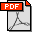Pankaj K. Agarwal, Jie Gao, Leonidas Guibas, Haim Kaplan, Vladlen Koltun, Natan Rubin and Micha Sharir. Kinetic Stable Delaunay Graphs. 26th Annual ACM Sympoium on Computational Geometry, 2010. Abstract: The best known upper bound on the number of topological changes in the Delaunay triangulation of a set of moving points in R2 is (nearly) cubic, even if each point is moving with a fixed velocity. We introduce the notion of a stable Delaunay graph (SDG in short), a dynamic subgraph of the Delaunay triangulation, that is less volatile in the sense that it undergoes fewer topological changes and yet retains many useful properties of the full Delaunay triangulation. SDG is defined in terms of a parameter alpha > 0, and consists of Delaunay edges pq for which the (equal) angles at which p and q see the corresponding Voronoi edge e are at least alpha. We prove several interesting properties of SDG and describe two kinetic data structures for maintaining it. Both structures use O*(n) storage. They process O*(n2) events during the motion, each in O*(1) time, provided that the points of P move along algebraic trajectories of bounded degree; the O*(.) notation hides multiplicative factors that are polynomial in 1/alpha and polylogarithmic in n. The first structure is simpler but the dependency on 1/alpha in its performance is higher. Bibtex: ```@inproceedings{aggkkrs-ksdg-10, author = {Pankaj K. Agarwal and Jie Gao and Leonidas Guibas and Haim Kaplan and Vladlen Koltun and Natan Rubin and Micha Sharir}, title = {Kinetic Stable Delaunay Graphs}, booktitle="Proc. of the 26th ACM Symposium on Computational Geometry (SoCG'10)", year = {2010} } ```